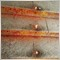# One order in a time213

hi everyone,

I have problem with my code,

How to make this open sell code only open one order at a time until it close by hit TP or SL then will continue to open another order.

Thanks.

extern   double StopLoss = 200;     // StopLoss

extern   double TakeProfit = 100;   //Takeprofit

extern   double LotSize = 0.1;        //LotSize

extern   int Slippage = 3;

int start()

{

//Opening SELL

double SL = Bid + (StopLoss*Point);

double TP = Bid - (TakeProfit*Point);

double Lot = LotSize;

int ticket = OrderSend (Symbol(),OP_SELL,Lot,Bid,Slippage,SL,TP,NULL,0,0);20093
eevviill7

Count opened orders.

if(OrdersTotal()==0)...213

Vasyl Nosal:

Count opened orders.

if(OrdersTotal()==0)...

Thanks, its work. do you know where i can refer all this things with some example.3860

zaharulrizal:

hi everyone,

I have problem with my code,

How to make this open sell code only open one order at a time until it close by hit TP or SL then will continue to open another order.

Thanks.

extern   double StopLoss = 200;     // StopLoss

extern   double TakeProfit = 100;   //Takeprofit

extern   double LotSize = 0.1;        //LotSize

extern   int Slippage = 3;

int start()

{

//Opening SELL

double SL = Bid + (StopLoss*Point);

double TP = Bid - (TakeProfit*Point);

double Lot = LotSize;

int ticket = OrderSend (Symbol(),OP_SELL,Lot,Bid,Slippage,SL,TP,NULL,0,0);

int ticket;

if(OrdersTotal()==0  && ---aditional conditions if any---)

{

ticket = OrderSend (Symbol(),OP_SELL,Lot,Bid,Slippage,SL,TP,NULL,0,0);

return;

}

Even those if opening more then one trades erase return . Best of luck.20093
eevviill7
zaharulrizal:
Thanks, its work. do you know where i can refer all this things with some example.
What things?